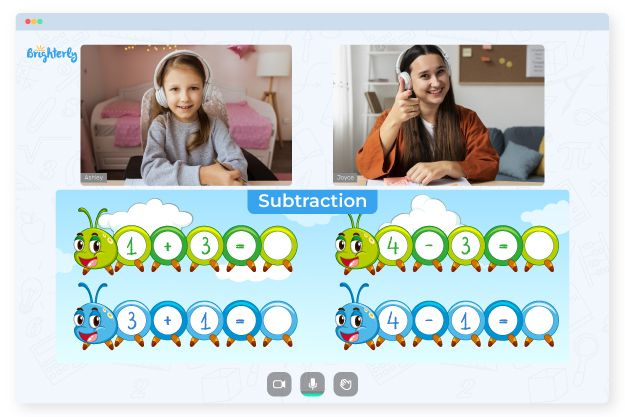# Subtraction Property of Equality – Definition with Examples

Are you ready to dive into the wonderful world of math and explore the fascinating concept of the Subtraction Property of Equality? If you’re a young math enthusiast looking to expand your knowledge, you’ve come to the right place at Brighterly! In this article, we will uncover the definition of the Subtraction Property of Equality, discuss its formula, provide verification techniques, and even tackle problems involving fractions. So let’s embark on this mathematical journey together.

## What is the Subtraction Property of Equality?

The Subtraction Property of Equality is a fundamental principle in mathematics that allows us to manipulate equations involving subtraction. Simply put, it states that if you subtract the same quantity from both sides of an equation, the two sides remain equal. This property is an extension of the more familiar Addition Property of Equality, which deals with addition operations.

## Subtraction Property of Equality Definition

The definition of the Subtraction Property of Equality is straightforward. Let’s say we have an equation: a – b = c. According to the Subtraction Property of Equality, if we subtract b from both sides of the equation, the equation remains balanced. Therefore, we obtain: a – b – b = c – b, which simplifies to a – 2b = c – b.

## Subtraction Property of Equality Formula

To summarize the Subtraction Property of Equality in formulaic terms, we can express it as follows:

If a = b, then a – c = b – c.

This formula illustrates that we can subtract the same quantity (c) from both sides of an equation (a and b) while preserving their equality.

## Verification of Subtraction Property of Equality

You might be wondering, “How can we verify the Subtraction Property of Equality?” Well, it’s quite simple. Let’s consider an equation: 6 – 3 = 9 – 6. By applying the Subtraction Property of Equality, we can subtract 3 from both sides of the equation, resulting in 6 – 3 – 3 = 9 – 6 – 3. Simplifying further, we have 6 – 6 = 9 – 9, which is true since both sides of the equation equal zero. Thus, we have successfully verified the Subtraction Property of Equality.

## Subtraction Property of Equality Including Fractions

The Subtraction Property of Equality is not limited to whole numbers; it extends to fractions as well. Let’s examine an example: 2/3 – 1/3 = 4/6 – 2/6. By subtracting 1/3 from both sides of the equation, we get 2/3 – 1/3 – 1/3 = 4/6 – 2/6 – 1/3. Simplifying, we obtain 2/3 – 2/3 = 4/6 – 3/6, which equals zero on both sides. Hence, the Subtraction Property of Equality remains valid even when dealing with fractions.

## Practice Problems on Subtraction Property of Equality

To reinforce your understanding of the Subtraction Property of Equality, let’s tackle a few practice problems:

1. Solve the equation: x – 7 = 15.
2. If a – b = c, find the value of b in the equation 2a – 3b = 5c.
3. What is the solution to the equation: 4 – (2x – 5) = 1 + (3x – 2)?

Remember to apply the Subtraction Property of Equality correctly to arrive at the solutions. If you need further guidance, feel free to check out our detailed explanations and step-by-step solutions.

## Conclusion

Congratulations! You have now grasped the Subtraction Property of Equality and its various applications. This essential mathematical principle empowers young minds to confidently manipulate equations involving subtraction, fostering a strong foundation in mathematics.

At Brighterly, our mission is to make learning math enjoyable and accessible for children. We believe that by understanding concepts like the Subtraction Property of Equality, young learners can develop problem-solving skills and critical thinking abilities that will serve them well throughout their mathematical journey and beyond.

## Frequently Asked Questions on Subtraction Property of Equality

### What is the Subtraction Property of Equality?

The Subtraction Property of Equality is a fundamental principle in mathematics that allows us to manipulate equations involving subtraction. It states that if you subtract the same quantity from both sides of an equation, the two sides remain equal. This property is a powerful tool that helps us solve equations and understand the relationship between different values.

### Can the Subtraction Property of Equality be applied to fractions?

Absolutely! The Subtraction Property of Equality extends to fractions as well. When working with fractions, you can subtract the same fraction or common denominator from both sides of an equation to maintain equality. It’s important to remember to simplify and adjust fractions accordingly to keep the equation balanced.

### How can I verify the Subtraction Property of Equality?

Verifying the Subtraction Property of Equality is relatively straightforward. To do so, you can choose an equation and perform the same subtraction operation on both sides. If the resulting equation remains balanced, with both sides still equal, then the Subtraction Property of Equality holds true. This verification process helps build confidence in the principle and reinforces its validity in various mathematical scenarios.

### Are there any limitations to the Subtraction Property of Equality?

The Subtraction Property of Equality is a versatile and robust principle that has no inherent limitations. However, it’s essential to follow the correct steps and apply it accurately in equation manipulations. Care should be taken when dealing with complex equations, as multiple operations may be involved. It’s crucial to perform each step correctly and maintain the balance of the equation throughout the process.

Sources:

Struggling with Subtraction?• Is your child finding it challenging to understand subtraction lessons?
• An online tutor could be the solution.

Does your child struggle to master subtraction lessons? Try lessons with an online tutor.